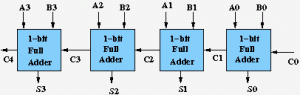# GATE | GATE-CS-2015 (Set 2) | Question 65

A half adder is implemented with XOR and AND gates. A full adder is implemented with two half adders and one OR gate. The propagation delay of an XOR gate is twice that of an AND/OR gate. The propagation delay of an AND/OR gate is 1.2 microseconds. A 4-bit ripple-carry binary adder is implemented by using full adders. The total propagation time of this 4-bit binary adder in microseconds is
(A) 19.2 microseconds

```Let us first calculate propagation delay of a single

Propagation Delay by n bit full adder is (2n + 2)
gate delays.
[See this for formula].

Here n = 1, so total delay of a 1 bit full adder
is (2 + 2)*1.2 = 4.8 ms

Delay of 4 full adders is = 4 * 4.8 = 19.2 ms```
My Personal Notes arrow_drop_up
Article Tags :

1

Please write to us at contribute@geeksforgeeks.org to report any issue with the above content.